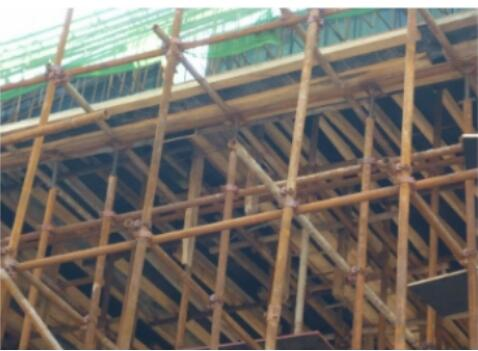# 企业负责人A类题库

A.2m(含2m)

B.3m(含3m)

C.4m(含4m)

D.5m(含5m)

A.允许

B.无所谓

C.禁止

D.看情况

A.分类型集中堆放整齐

B.集中堆放

C.就地焚烧

D.无

A.管制

B.警告

C.行政拘留

D.罚金

A.安全管理人员协议

B.安全质量保证书

C.安全生产协议书

D.安全责任区域划分

A.12m

B.16m

C.20m

D.24m

A.10％

B.20％

C.30％

D.40%

A.18m

B.20m

C.24m

D.30m

A.绝缘鞋

B.防滑鞋

C.高跟鞋

D.帆布鞋

A.单层防护棚

B.单层防护网

C.双层防护网

D.顶部能防止穿透的双层防护廊

A.链轮

B.手拉链

C.齿轮传动装置

D.起重链解析

A.宪法

B.法律

C.宪法或法律

D.法律及行政法规

A.建筑施工企业

B.建设单位

C.监理单位

D.主管部门

A.1

B.1.1

C.1.2

D.1.4

A.允许

B.禁止

C.应设专人监控

D.采取措施后可以

A.不承担责任

B.承担连带责任

C.不承担连带责任

D.承担责任

A.导线

B.支撑物

C.零线

D.地线

A.政府要求的工期

B.合同约定的工期

C.监理单位建议的工期

D.施工单位计算的工期

A.每季至少进行一次

B.每月至少进行一次

C.每年至少进行一次

D.每年至少进行二次

A.一

B.二

C.三

D.六

A.一次

B.两次

C.三次

D.四次

A.15

B.20

C.30

D.40

A.1

B.2

C.3

D.4

A.14d

B.28d

C.30d

D.40d

A.安全检查

B.安全管理

C.监督检查

D.安全指导

A.可以接头

B.严禁接头

C.错开接头

D.没有规定

A.保障.限位

B.担保.限位

C.保险.限量

D.保险.限位

A.遵照国家有关规定执行

B.由市行业主管行政部门确定

C.由区县政府或其指定的有关部门确定

D.由乡镇政府或其指定的有关部门确定

A.36

B.24

C.12

D.9

A.《建筑法》

B.《安全生产法》

C.《建筑工程安全生产管理条例》

D.《建筑工程质量管理条例》

A.室内

B.室外

C.窗

D.窗外

A.偶然损失原则

B.安全第一原则

C.因果关系原则

D.动态相关原则

A.东平建筑公司

B.原北二建

C.大政监理公司

D.原北市市政府

A.防护用品

B.福利用品

C.专供用品

D.保护用品

A.不得

B.应该

C.可以

D.需要解析

A.民事责任

B.刑事责任

C.行政责任

D.赔偿责任

A.建设工程质量监督机构

B.工程监理单位

C.安全监督机构

D.建设主管部门

A.建设行政主管部门

B.颁发资质证书的机关

C.中国建筑业协会

D.国务院

A.20cm

B.25cm

C.30cm

D.35cm

A.内电线路

B.光缆

C.输电线路

D.外电线路

A.日常隐患排查

B.综合性隐患排查

C.专项隐患排查

D.事故类比隐患排查

A.使用危险报告书

B.收集职工建议

C.使用安全反馈程序

D.计划.量测.总结和评审安全工作

E.建立并完善企业的安全管理政策和组织结构

A.马上报警

B.施工单位应当采取措施防止事故扩大

C.需要移动现场物品时，应当做出标记和书面记录，妥善保管有关证物

D.保护事故现场

E.施工单位不许采取任何措施

A.时间

B.事故单位

C.地点

D.伤亡人数

E.赔偿数额

A.降级

B.撤职

C.并处本人上一年60%—100%的罚款

D.并处本人上一年40%—80%的罚款

E.并处本人上一年30%—80%的罚款

A.架体高度不应大于4倍楼层高

B.架体高度不应大于5倍楼层高

C.架体高度不应大于1.2m

D.架体高度不应大于1.5mA.梁底中部支撑立杆上部可调支托螺杆外伸长度超过200mm

B.外侧边梁底部立杆小横杆未设置不到位

C.梁底中部支撑立杆可调支托上部未设置双钢管，造成立杆偏心受力

D.梁底立杆纵向水平拉杆设置不到位

E.梁底支撑架体部分连接扣件螺栓未坚固到位

A.合法

B.公正

C.及时

D.公开

E.便民

A..基坑支护

B..模板工程

C..钢筋工程

D..砼工程

E..起重吊装作业

A.安全生产责任制度

B.安全生产资金保障制度

C.安全生产教育培训制度

D.安全督查和处罚制度

E.安全生产事故与报告与处理制度。

A.建设主管部门

B.公司(分公司)级

C.工地(项目部)级

D.安全监督部门

E.班组级

A.编制拆装方案

B.停止其他工种人员作业

C.企业负责人参加并监督

D.制定安全施工措施

E.由专业技术人员现场监督

A.施工工艺

B.操作方法

C.操作要求

D.安全质量要求

E.保障工程安全质量的主要措施

A.坚持“有法必依.执法必严.违法必究”的原则

B.坚持以事实为依据,以法律为准绳的原则

C.坚持预防为主的原则

D.坚持行为监察与技术监察相结合的原则

E.坚持监察与服务相结合的原则

A.工作服

B.防滑鞋心

C.佩戴安全帽

D.安全带

A.明确各自的安全生产管理职责

B.明确各自应当采取的安全措施

C.明确各自应当采取的质量措施

D.指定各自项目管理人员进行安全检查与协调

E.指定各自专职安全生产管理人员进行安全检查与协调

A.民间组织

B.军队.警察

C.法庭

D.社会团体

E.监狱

A.机械性噪声

B.施工人员叫喊声

C.空气动力性噪声

D.临街面的嘈杂声

E.电磁性噪声

A.钢材

B.木材

C.铝合金

D.竹材

E.铜材

A.轻型井点

B.喷射井点

C.管井井点

D.深井泵

E.由渗井点

A.正确

B.错误

A.正确

B.错误

A.正确

B.错误

A.正确

B.错误

A.正确

B.错误

A.正确

B.错误

A.正确

B.错误

A.正确

B.错误

A.正确

B.错误

A.正确

B.错误

A.正确

B.错误

A.正确

B.错误

A.正确

B.错误

A.正确

B.错误

A.正确

B.错误

A.正确

B.错误

A.正确

B.错误

A.正确

B.错误

A.正确

B.错误

A.正确

B.错误

A.正确

B.错误

A.正确

B.错误

A.正确

B.错误

A.正确

B.错误

A.正确

B.错误

A.正确

B.错误

A.正确

B.错误

A.正确

B.错误

A.正确

B.错误

A.正确

B.错误

A.正确

B.错误

A.正确

B.错误

A.正确

B.错误

A.正确

B.错误

A.正确

B.错误

A.正确

B.错误

A.正确

B.错误

A.正确

B.错误

A.正确

B.错误

A.正确

B.错误